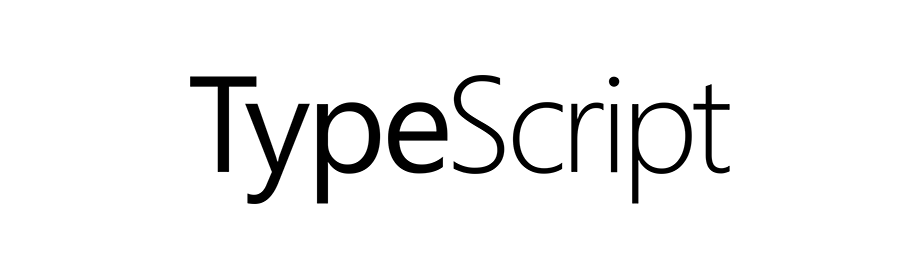# TypeScript - Functions

TypeScriptIn this tutorial we will learn to work with functions in TypeScript.

In JavaScript if we want to create a function that will print "Hello World" we write the following code.

``````function greetings() {
console.log("Hello World");
}

// calling
greetings();
``````

The above code is also valid in TypeScript and it will also give us the same result.

Now we will add types and enhance the functions.

## Function with no return type

If a function is not going to return any value then we can set the return type to `void`.

``````function greetings(): void {
console.log("Hello World");
}

// calling
greetings();
``````

## Function returning some value

Following is the syntax of a function returning value.

``````function funName(): type {
// some code...
}
``````

Where, `funName` is the name of the function and it returns a value of given `type`.

In the following example the function `getNumber` returns value of type `number`.

``````function getNumber(): number {
return Math.random();
}

// calling
console.log("Random number: " + getNumber());
``````

## Function with parameters

Following is the syntax of a function with parameters.

``````function funName(val1: type): retType {
// some code...
}
``````

Where, `funName` is the name of the function. `val1` is a parameter of given `type`. And the return type of the function is `retType`.

In the following example we have an `addition` function which takes two numbers as argument and returns a number.

``````function addition(x: number, y: number): number {
return x + y;
}

// calling
console.log("Sum: 1 + 2 = " + addition(1, 2));
``````

Note! If we pass value that is not of `number` type to the above `addition()` function then we will get an error.

## Function type

We can use function signature (parameter list and return type) to create a new type.

In the following example we are creating a variable `myFun` of function type using Arrow Function.

``````let myFun: (v1: number, v2: number) => number;
``````

So, the above line tells us that `myFun` can only be assigned a function that has two parameters of type `number` and the return type of the function isnumber.

So, lets create a function and then assign it to the `myFun` variable.

``````// function
function multiply(x: number, y: number): number {
return x * y;
}

// variable of function type
let myFun: (v1: number, v2: number) => number;

// assign function
myFun = multiply;

// now call the function
console.log("Multiply 1 x 2 = " + myFun(1, 2));
``````